Latest Banking jobs   »   IBPS quant

# Quantitative Aptitude Quiz For IBPS PO Prelims 2023 -17th September

Q1. In a bag, there are 16 balls of three different colors i.e. red, blue and green. Number of red and blue balls is 9 and difference between red and green ball is 4 then find the probability of getting a ball of each color if three balls are picked at random?
(a) 5/28
(b) 4/27
(c) 7/36
(d) 8/35
(e) 9/40

Q2. A bag contains only two colour balls i.e. red & green. Ratio of number of green balls to red balls is 4 : 3 is four green balls taken out from the bag, then the new ratio of green balls to red balls become 8 : 9. Find the probability of that if two balls taken out from the bags and both balls will be same colour?
(a) 17/35
(b) 17/38
(c) 14/35
(d) 12/35
(e) 11/35

Q3. A bag contains x green balls, 7 blue balls and 8 red balls. When two balls are drawn from bag randomly, then the probability of one ball being green and one ball being red is 4/15. Find value of x (number of green balls cannot be more than 18 balls).
(a) 3
(b) 5
(c) 10
(d) 9
(e) 15

Q4. In a bag, there are some red and total 9 black and yellow balls. Probability of selecting two red balls from that bag is 1/7 which is 250% of the probability of selecting two black balls. Find number of yellow balls in that bag if the number of black balls is even.
(a) 3
(b) 5
(c) 6
(d) 4
(e) None of these

Q5. An urn contains 4 red, 5 green, 6 blue and some yellow balls. If two balls are drawn at random, the probability of getting at least one yellow ball is 17/38. find the yellow balls in the urn.
(a) 4
(b) 5
(c) 6
(d) 10
(e) 15

Q6. An urn contains 6 red balls and 9 green balls. Two balls are drawn from the urn one after other without replacement. Find the probability of drawing a red ball when a green ball has been drawn from the urn.
(a) 3/15
(b) 3/7
(c) 9/14
(d)7/11
(e) 2/5

Q7. Bag-A contains 6 blue balls, 7 red balls and 2 green balls and Bag-B contains 5 blue balls, x red balls and 2 green balls. A bag is chosen randomly, and two balls are taken out from it randomly, then the probability of getting two red balls is 2/15. Find number of red balls in Bag-B.
(a) 2
(b) 5
(c) 1
(d) 4
(e) None of the above.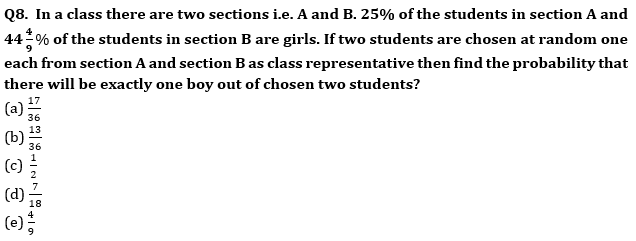Q9. The sum of area of a circle & area of a rectangle is equal to 2136 sq. cm. The diameter of the circle is 42 cm. What is the difference between the circumference of the circle & the perimeter of the rectangle, if the breath of the rectangle is 30 cm?
(a) 42 cm
(b) 22 cm
(c) 30 cm
(d) 11 cm
(e)18 cm

Q10. A solid is in the form of a cylinder with hemispherical ends. If the height of the solid is 26cm and diameter of cylindrical part is 14cm, then find the cost of covering the solid with metal sheet (Cost of metal sheet is Rs.15/cm2).
(a) Rs.16570
(b) Rs.15440
(c) Rs.17160
(d) Rs.12420
(e) Rs.14370

Solutions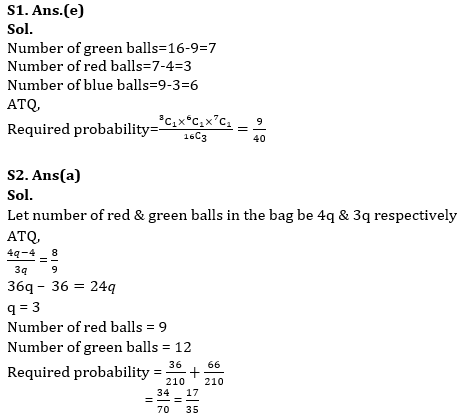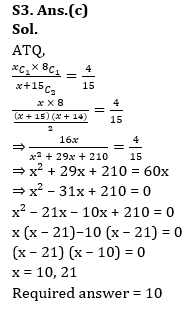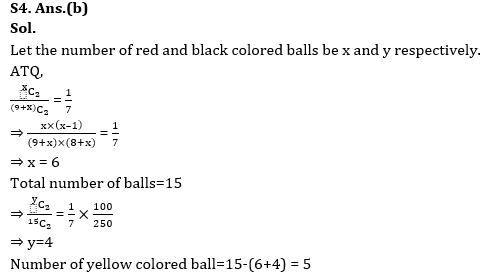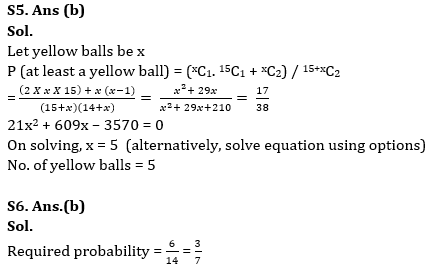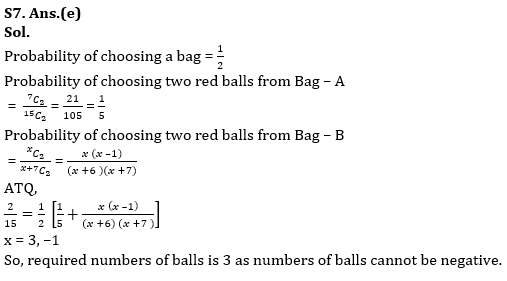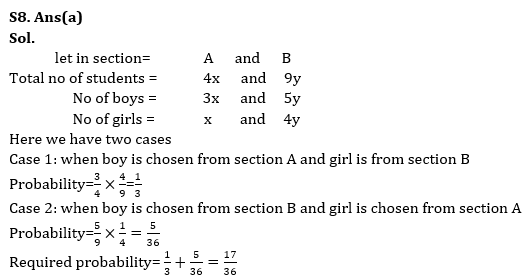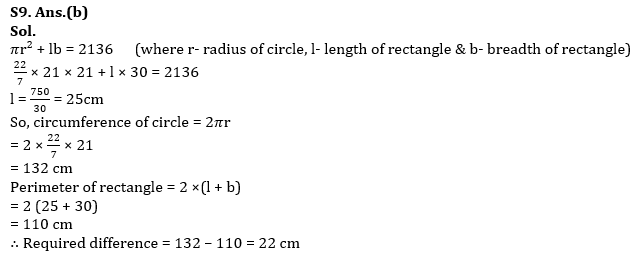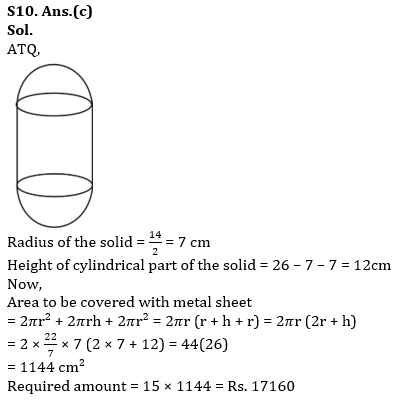. .

## FAQs

### When will the IBPS PO prelims 2023 be conducted?

IBPS PO Prelims will be conducted on 23, 30 September, and 1 October 2023.

#### Congratulations!Union Budget 2023-24: Free PDF